﻿ Hydrogeologist workbench (ANSQUICK) > Lugeon tests

# Lugeon (packer) tests

Navigation:  Hydrogeologist workbench (ANSQUICK) >

# Lugeon (packer) tests

ANSDIMAT offers interpretation of packer tests by the Lugeon method, where hydraulic conductivity is calculated from pressure and injection rate measurements.

The dialog window "Lugeon tests" is opened by selecting menu "File > Hydrogeologist workbench > Lugeon test".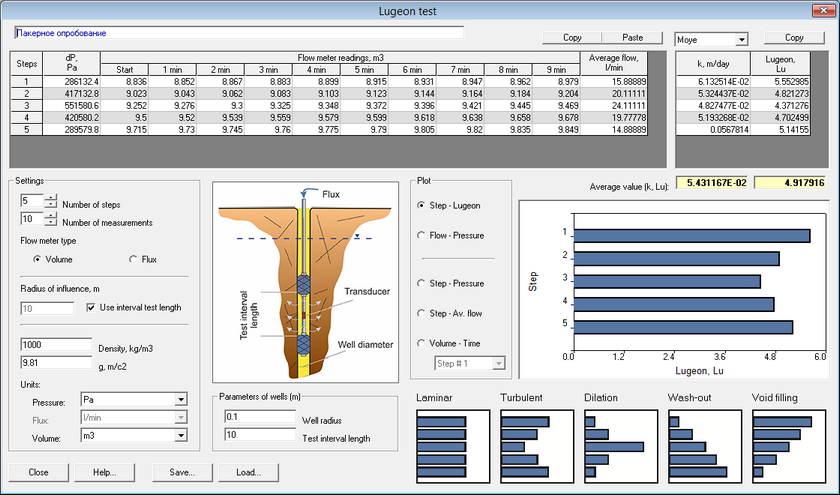Example of dialog window "Lacker test" - data analysis for test stages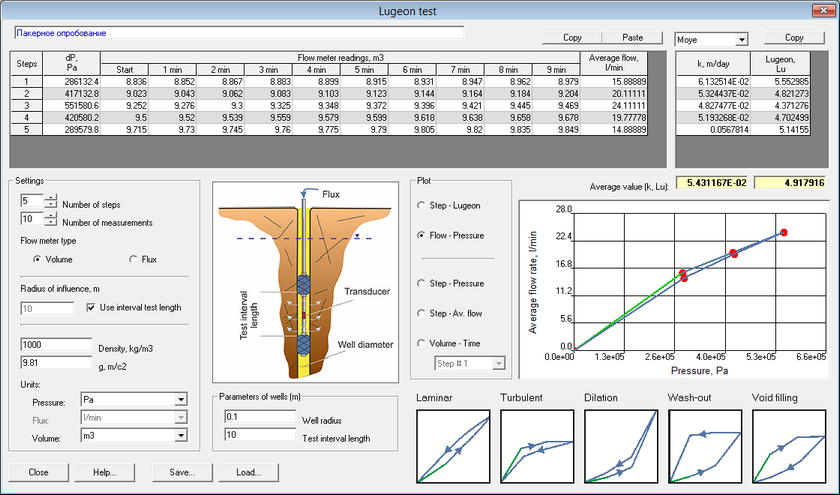Example of dialog window "Lugeon test" - data analysis for inection rate vs pressure plots

Before the start of a test, the maximum testing pressure Pmax is determined based on the depth of the borehole screen.

The testing process aims at sustaining a constant pressure by injecting water volumes and measuring both, pressure and water flow. The standard procedure for packer testing is explained below:

1.Pressure is changed by steps from the lowest to highest pressure as: 0.5Pmax, 0.75Pmax, Pmax, 0.75Pmax, 0.5Pmax.

2.Constant pressure P is maintained for 10 minutes at each step.

3.The water injection rate Q, required to sustain pressure, is measured every minute.

4.The Lugeon value (Lu) is calculated for each step by the formula: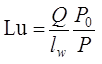where Q – average injection rate for sustaining constant pressure, l/min, lw –  length of testing interval, m; P – constant pressure at each step, Pa. P0 = 1 MPа – scaling pressure coefficient.

5.Lugeon values are plotted as a bar diagram for each pressure step. Depending on the shape of the plot, hydrodynamic conditions during the test fall into one of the five categories: 1) laminar flow; 2) turbulent flow; 3) opening of fractures; 4) washing out fine material; and 5) filling in voids/fractures.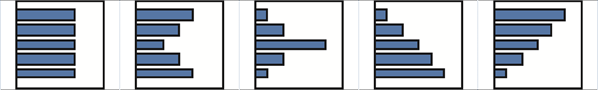6.Water injection rates are plotted against pressure values. Depending on the shape of the plot, hydrodynamic conditions during the test fall into one of the five categories: 1) laminar flow; 2) turbulent flow; 3) opening of fractures; 4) washing out fine material; and 5) filling in voids/fractures.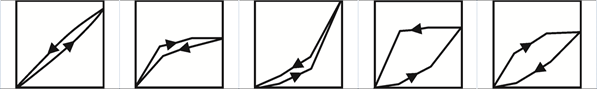Arrows show the directions of pressure changes from step to step.

7.For each step, the hydraulic conductivity is calculated using one of the three methods below:

– Moye formula: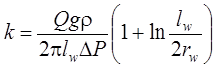– Thiem formula: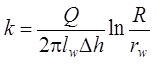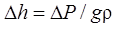where ΔP – borehole pressure change , Pa; g – gravity acceleration value, m/s2; ρ – density of injected water, kg/m3.

– Lugeon formula:

1 Lu 1.3∙10-7 m/s 0.011 m/d.

Fracturing can be qualitatively described based on mean lugeon and hydraulic conductivity values.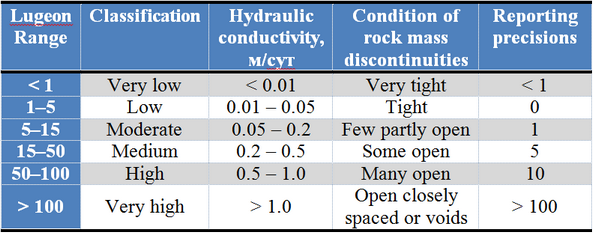Explanatory table

 Field on the window top Title (description) of packer tests Table on the left The table contains factual measurements of pressure and injection rate for each step of the test First column - N of step Second column - The constant pressure, that is maintained during the corresponding step. Units of measurements can be selected from the drop-down menu "Pressure" The next columns contain injection rates or volume of water injected during the corresponding stage at different time intervals. The number of columns depends on the number of measurements (time intervals). The user can choose between entering flux or volume by checking one of the "Flow meter type" items. For the "Volume" option, the first measurement (Column "Start") corresponds to the measured volume before the start of the test, and the next columns corresponds to volume measurements after 1 min, 2 min etc. For the "Flux" option, the columns correspond to the injection rate measured after 1 min, 2 min etc. The last column automatically calculates the average injection rate over the entire step length. Measurement units for flux or volume can me selected from the relevant drop-down menus. Button "Copy" Copies table data on the Clipboard Button "Paste" Pastes data from the Clipboard into the table starting from the cursor location Table on the right The table contains hydraulic conductivity values (left column) and lugeon values (right column) Drop-down menu above the table Selection of calculation formula for hydraulic conductivity. ANSDIMAT uses the Moye, Thiem and Lugeon methods for calculations. After the method is changed from the drop-down menu, hydraulic conductivity values are recalculated automatically Button "Copy" Copies table data on the Clipboard Fields "Average value (k, Lu)" Calculation of average values of hydraulic conductivity (m/day) and lugeon parameter (Lu). The parameters are calculated based on the values from the table on the right Frame "Settings" Selection of number of steps, number of measurements and units of measurements Field "Number of steps" Quantity of steps with constant pressure: between 5 and 99 Field "Number of measurements" Quantity of measurements of injection rate/volume in one step: between 3 and 99 Choice options "Flow meter type" Selects whether flux or volume of injected water were measured for inputs on the table on the left Option "Volume" Volume of injected water recorded during the test Option "Flux" Flux (injection rate) of water recorded during the test Text box "Radius of influence" Assumed radius of influence for the test. An option is offered to assign test interval as radius of influence. When this option is not selected (the relevant box is unchecked), the radius of influence is entered manually Field "Density" Density of injected water. This field is used for converting injection rate in l/min units and for calculations of hydraulic conductivity Field "g, m/c2" Gravity acceleration coefficient = 9.8 m/s2. This value is used for converting pressure in hydraulic head in calculations of hydraulic conductivity List "Units" Drop-down menus allows selection of measurement units for pressure, flux and volume of injected water List "Pressure" Selection of pressure measurement units List "Flux" Selection of flux measurement units List "Volume" Selection of volume measurement units Frame "Parameters of well" Borehole radius and test interval Field "Well radius" Enters the radius of the tested borehole (m) Field "Test interval length" Enters tested interval (m) Frame "Plot" Choice of plots for visual analysis of hydrodynamic conditions during the test Option "Step - Lugeon" Diagram of lugeon values for the test steps (2-nd column of the right table). This plot is used to characterize the test conditions (i.e. laminar or turbulent flow) Option "Flow - Pressure" Plot of step-averaged injection rate (last column of the left table) vs pressure of injected water (2-nd column of the left table). This plot is used to characterize the  test conditions (i.e. laminar or turbulent flow) Option "Step - Pressure" Diagram of pressure variations for the test steps (2-nd column of the left table) Option "Step - Average Flow" Diagram of average flow variations for the test steps (last column of the left table) Option "Volume - Time" or "Flux - Time" Hydrograph of flow rate or injected water volume during each the test. A type of plot depends on whether options "Flux"or "Volume"are selected. Step can be selected from the drop-down list. Drop-down list Selection of test step for plotting Flux/Volume hydrograph Button "Save" Saves lugeon test data from the current dialog window in an internal ANSDIMAT format (file with extension ATL) Button "Load" Loads file with saved lugeon test data (file with extension ATL)